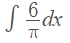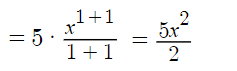# Constant Rule of Integration

The constant rule of integration tells you how to find an integral for a constant quantity like 7, ⅓ or π. The rule is defined as:

a dx = ax.

## Constant Rule of Integration Examples

Example problem #1: Use the constant rule of integration to evaluate the indefinite integral y = ∫ 4 dx.

Step 1: Place the constant in the question into the rule:
4 dx → 4x.

The solution is 4x + C.

Example problem #2: Evaluate the indefinite integral ∫ ½ dx.

Step 1: Place the constant into the rule:
½ dx½.

Step 2: Add a “+ C”:
The solution is ½x + C.

Example problem #3: Evaluate the following:Step 1: Place the constant into the rule:
= (6/π)x.

Step 2: Add a “+ C”:
The solution is = (6/π)x + C.

Notice that in the above problem π is a constant, so you can use the constant rule of integration. Euler’s number e is also a constant, so you can use this rule. However, ex is not a constant because of the x. However, the integral of ex is itself: ex + C.

## Constant Multiple Rule

The constant multiple rule for integrals is similar, but this time we’re concerned with finding an integral for a function multiplied by a constant:

The integral of ∫c f(x) dx is cf(x) dx.

They are almost exactly the same: the constant is brought out in front of the integral. In the case of the constant rule of integration though, that process only leaves “dx”, which is converted to x in our integral. With the constant multiple rule there is still a function f(x) to evaluate after you’ve used the constant multiple rule.

Example problem #4: Evaluate ∫5x dx.

Step 1: Pull the constant out in front:
5 ∫x dx.

Step 2: Integrate x with the power rule and simplify:Step 3: Add a + C:CITE THIS AS:
Stephanie Glen. "Constant Rule of Integration" From StatisticsHowTo.com: Elementary Statistics for the rest of us! https://www.statisticshowto.com/constant-rule-of-integration/